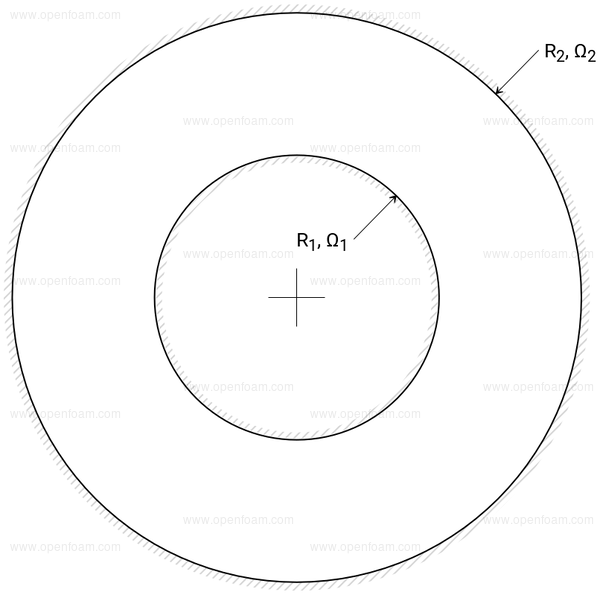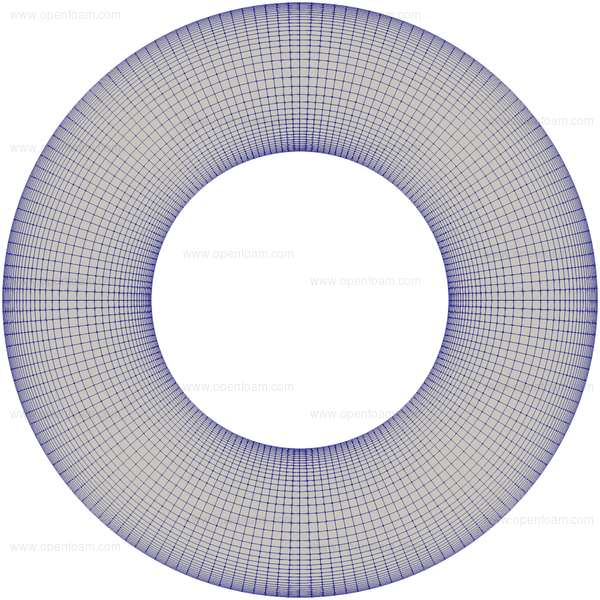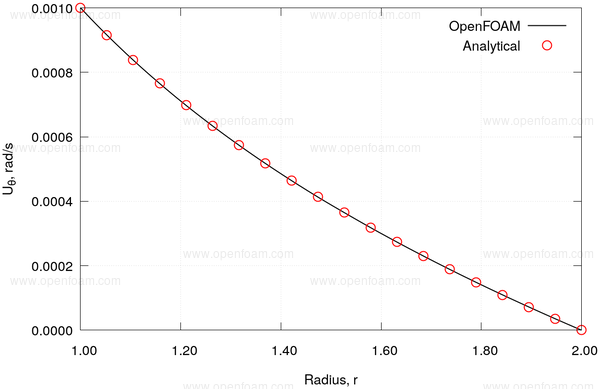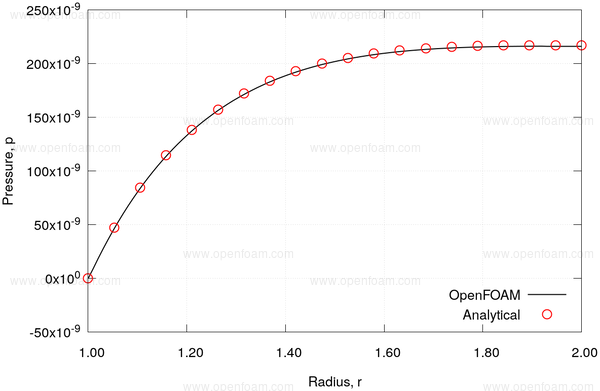## Overview🔗

• Solver: simpleFoam
• Incompressible
• Laminar
• Multiple Reference Frame (MRF)
• TODO: tutorial pathThe case comprises two cylinders, with inner radius $$R_1$$ rotating with angular velocity $$\Omega_1$$, and outer radius $$R_2$$ rotating with angular velocity $$\Omega_2$$.

The laminar case corresponds to a Reynolds number of 100. where the Reynolds number os defined as:

$Re = \frac{|\u_0| d}{\nu}$

Where $$\u_0$$ is the angular velocity of the inner cylinder, i.e.

$\u_0 = \Omega_1 R_1$

and $$d$$ is the distance between the cylinders, i.e. $$R_2 - R_1$$. Using an inner and outer radii of 1 and 2, respectively, and setting the kinematic viscosity to $$1$$, the angular velocity of the inner cylinder is 100 rad/s.

## Analytical solution🔗

Taylor  shows that the velocity, $$\u_{\theta}$$ is described by:

$\u_{\theta} = A r + \frac{B}{r}$

where $$A$$ and $$B$$ are constants defined as:

$A = \frac{\Omega_1 \left( 1 - \mu \frac{R_2^2}{R_1^2} \right)}{1 - \frac{R_2^2}{R_1^2}}$ $B = \frac{R_1^2 \Omega_1 (1 - \mu)}{1 - \frac{R_1^2}{R_2^2}}$

Here, $$\Omega_1$$ and $$\Omega_2$$ are the rotational speeds of the inner and outer cylinders, and

$\mu = \frac{\Omega_2}{\Omega_1}$

The steady flow equations for this case, in cylindrical co-ordinates reduces to

$\frac{1}{\rho}\frac{\partial p}{\partial r} - \frac{\u_{\theta}^2}{r} = 0$

On integrating with respect to radius an expression for the pressure is recovered:

$p = \frac{A^2 r^2}{2} + 2 A B \ln (r) + \frac{B^2}{2 r^2} + C$

## Mesh🔗## Boundary conditions🔗

• Outer cylinder fixed
• Inner cylinder rotates at a fixed angular velocity

## Results🔗

The rotational velocity, $$\u_\theta$$ can be directly reported during the calculation using a fieldCoordinateSystemTransform function object.